## Example Questions

### Example Question #5 : Tangent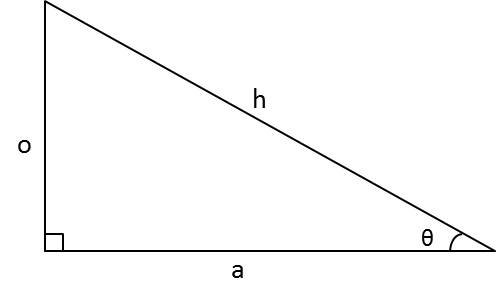For the above triangle,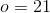and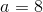. Find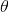.

This triangle cannot exist.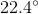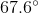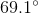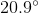Explanation:

With right triangles, we can use SOH CAH TOA to solve for unknown side lengths and angles. For this problem, we are given the opposite and adjacent sides of the triangle with relation to the angle. With this information, we can use the tangent function to find the angle.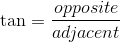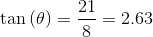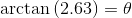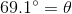### Example Question #6 : TangentIn the above triangle,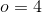and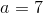. Find.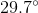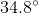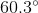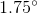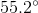Explanation:

With right triangles, we can use SOH CAH TOA to solve for unknown side lengths and angles. For this problem, we are given the opposite and adjacent sides of the triangle with relation to the angle. With this information, we can use the tangent function to find the angle.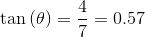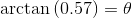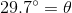### Example Question #7 : TangentFor the above triangle,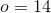and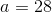. Find.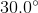This triangle cannot exist.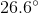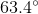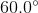Explanation:

With right triangles, we can use SOH CAH TOA to solve for unknown side lengths and angles. For this problem, we are given the opposite and adjacent sides of the triangle with relation to the angle. With this information, we can use the tangent function to find the angle.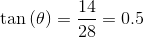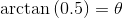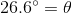### Example Question #8 : Tangent

A laser is placed at a distance of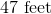from the base of a building that is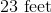tall. What is the angle of the laser (presuming that it is at ground level) in order that it point at the top of the building?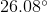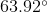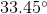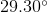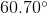Explanation:

You can draw your scenario using the following right triangle: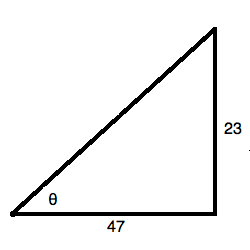Recall that the tangent of an angle is equal to the ratio of the opposite side to the adjacent side of the triangle. You can solve for the angle by using an inverse tangent function: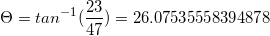or.

### Example Question #9 : Tangent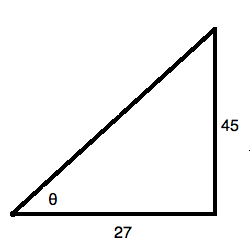What is the value of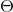in the right triangle above? Round to the nearest hundredth of a degree.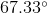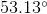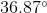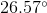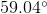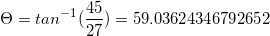or.